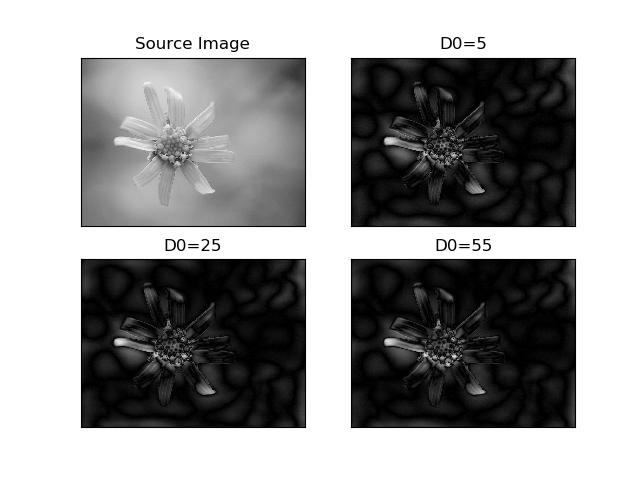2019-10-24 21:51:50 wujuxKkoolerter 阅读数 84
• ###### matlab信号处理和滤波去噪

739 人正在学习 去看看 刘昱显

# 01-图像降噪-平滑滤波-模板操作

## 1 模板操作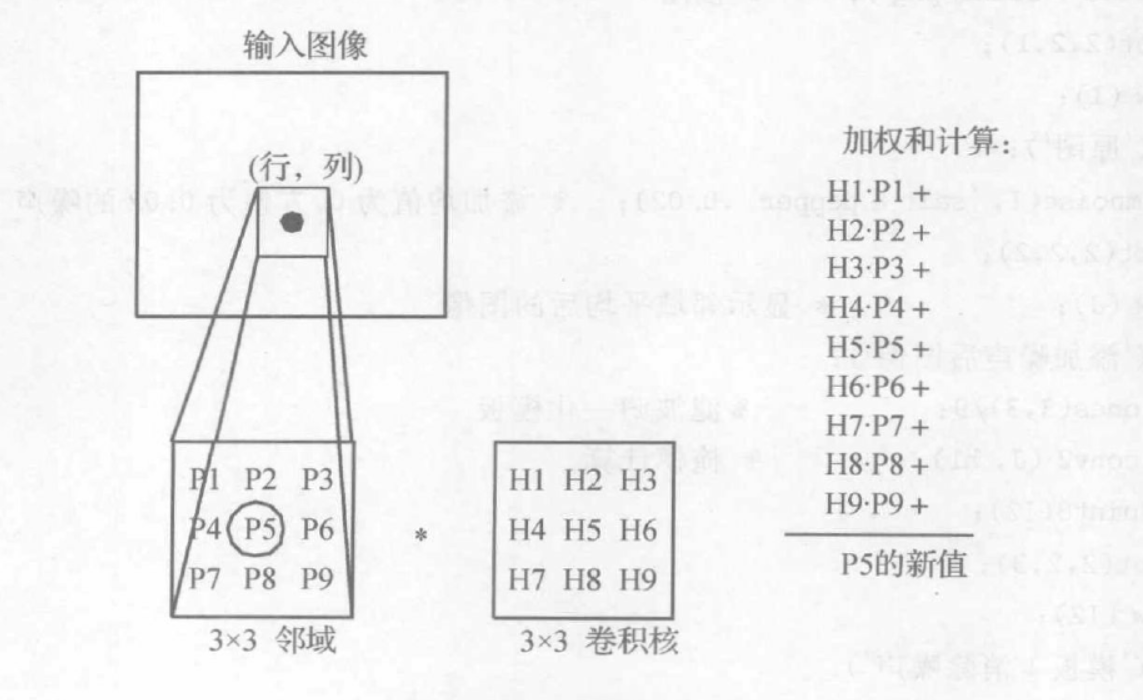## 2 掩模消噪法

$H_1 = \frac{1}{10} \begin{bmatrix} 1 & 1 & 1 \ 1 & 2 & 1 \ 1 & 1 & 1 \end{bmatrix}$，
$H_2 = \frac{1}{16} \begin{bmatrix} 1 & 2 & 1 \ 2 & 4 & 2 \ 1 & 2 & 1 \end{bmatrix}$，
$H_3 = \frac{1}{8} \begin{bmatrix} 1 & 1 & 1 \ 1 & 0 & 1 \ 1 & 1 & 1 \end{bmatrix}$

Python实现的代码如下：

import numpy as np
import scipy.signal as signal
%matplotlib inline
import matplotlib.pyplot as plt
import imageio

def convolve2d(img,fil,mode = 'same'):                #分别提取三个通道

if mode == 'fill':
h = fil.shape // 2
w = fil.shape // 2
img = np.pad(img, ((h, h), (w, w),(0, 0)), 'constant')
conv_b = _convolve2d(img[:,:,0],fil)              #然后去进行卷积操作
conv_g = _convolve2d(img[:,:,1],fil)
conv_r = _convolve2d(img[:,:,2],fil)

dstack = np.dstack([conv_b,conv_g,conv_r])      #将卷积后的三个通道合并
return dstack                                   #返回卷积后的结果

def _convolve2d(img,fil):

fil_heigh = fil.shape                        #获取卷积核(滤波)的高度
fil_width = fil.shape                        #获取卷积核(滤波)的宽度

conv_heigh = img.shape - fil.shape + 1    #确定卷积结果的大小
conv_width = img.shape - fil.shape + 1

conv = np.zeros((conv_heigh,conv_width),dtype = 'uint8')

for i in range(conv_heigh):
for j in range(conv_width):                 #逐点相乘并求和得到每一个点
conv[i][j] = wise_element_sum(img[i:i + fil_heigh,j:j + fil_width ],fil)
return conv

def wise_element_sum(img,fil):
res = (img * fil).sum()
if(res < 0):
res = 0
elif res > 255:
res  = 255
return res

src = imageio.imread('../../../../resources/images/f1.jpg')

kernel = np.array([[1.0,1.0,1.0],[1.0,2.0,1.0],[1.0,1.0,1.0]]) / 10.0


result = convolve2d(src,kernel,'fill')


plt.imshow(src)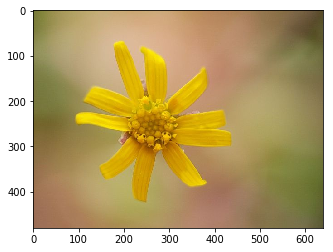plt.imshow(result)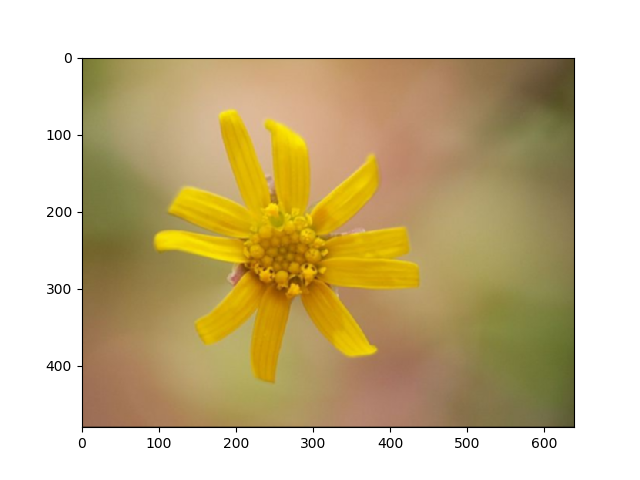2019-09-05 09:11:50 botao_li 阅读数 173
• ###### matlab信号处理和滤波去噪

739 人正在学习 去看看 刘昱显

#### 中值滤波去噪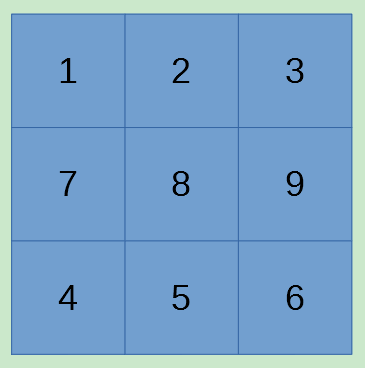$3\times 3$ 中值滤波为例，首先将图像数据送入 $3\times 3$ 二维缓冲，再将并行输出的 9 个像素点进行冒泡排序找到中值后输出：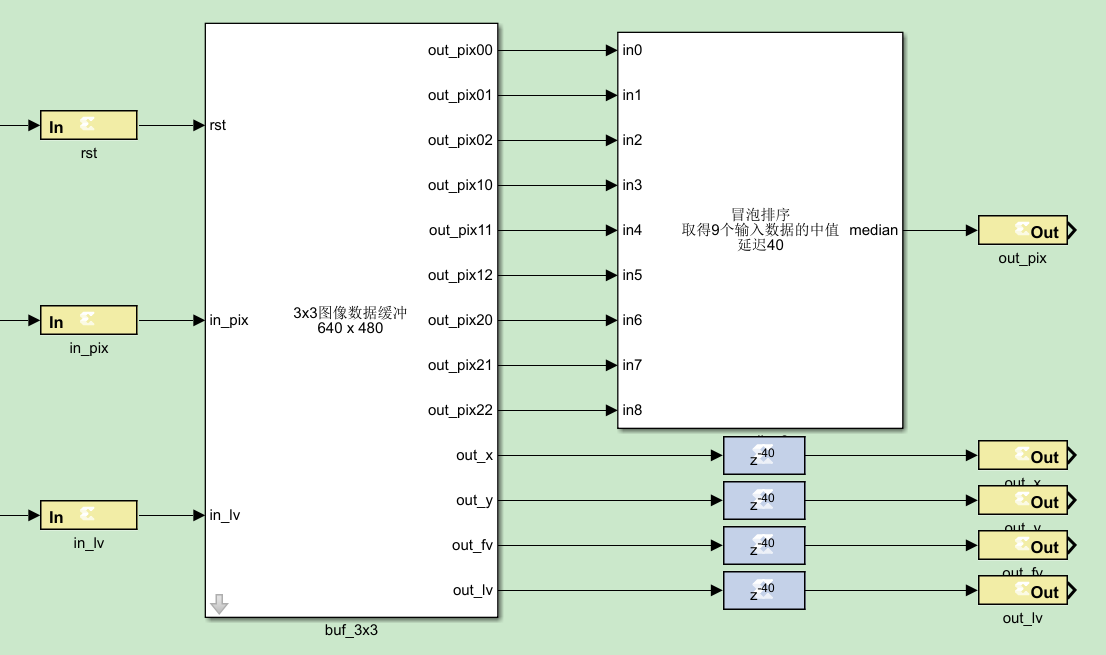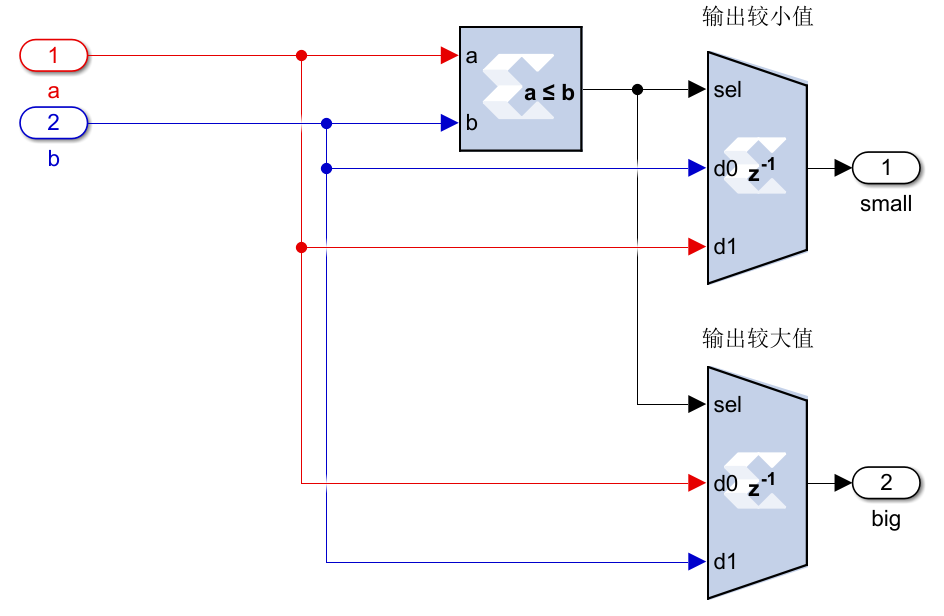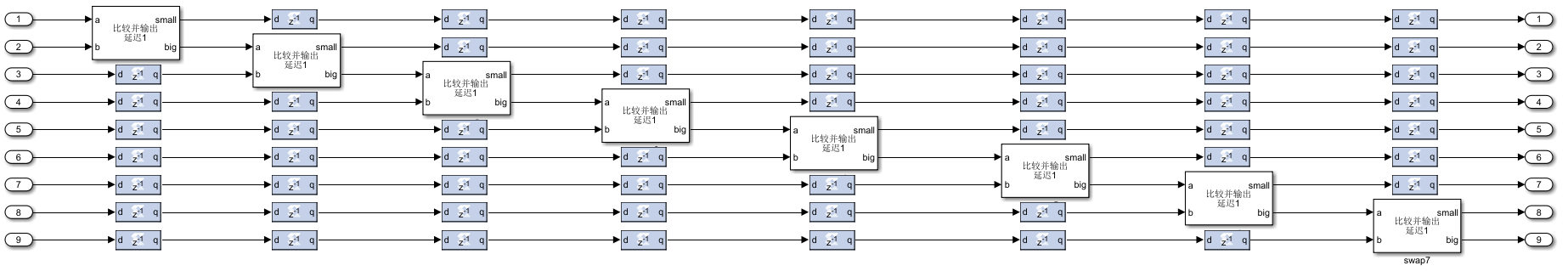5 个冒泡模块串联，5 轮冒泡之后在输出端口 5 找到中值 median：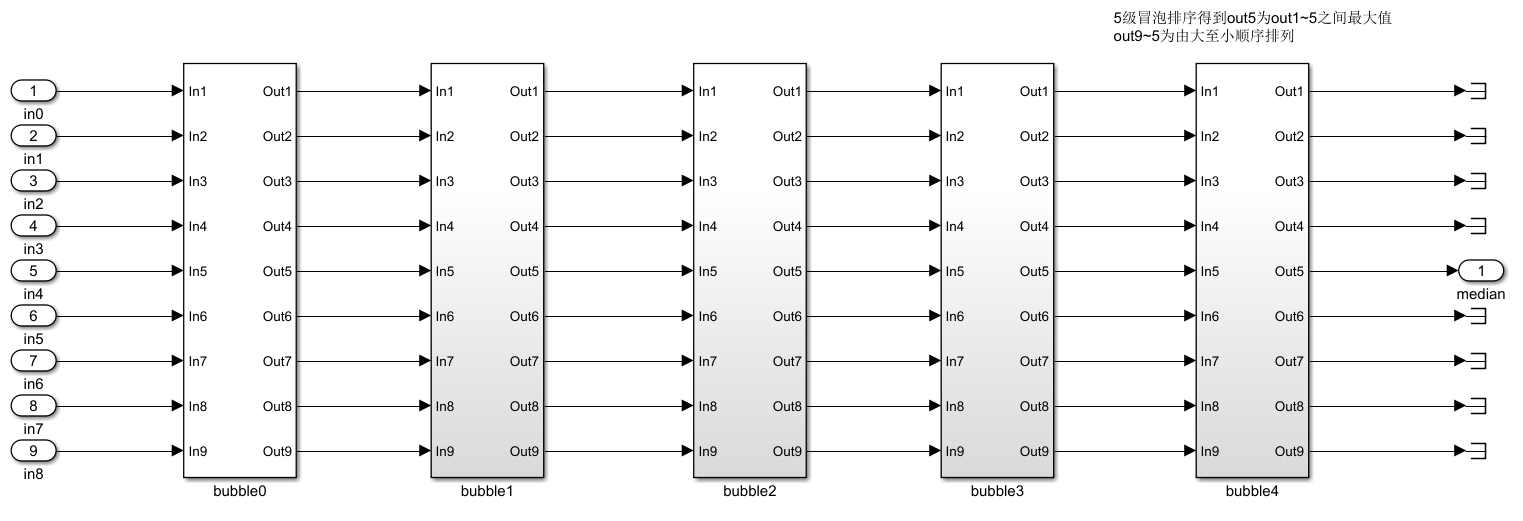2019-10-14 16:34:18 qq_24965393 阅读数 75
• ###### matlab信号处理和滤波去噪

739 人正在学习 去看看 刘昱显

1、方框滤波（盒式滤波）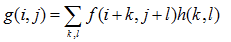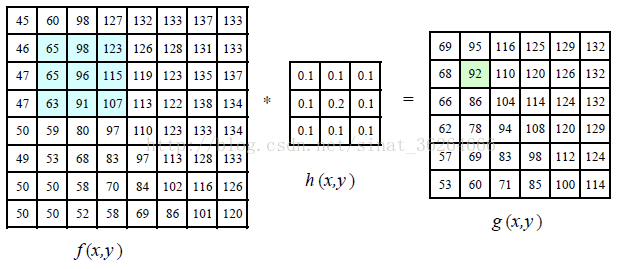OpenCV将盒式滤波封装在boxFilter()函数中，作用是输入一副图像对其进行盒式滤波。感兴趣的同学可以看看其源代码。下面来看下boxFilter()函数的定义：

void boxFilter( InputArray src, OutputArray dst, int ddepth, Size ksize,
Point anchor=Point(-1,-1), bool normalize=true, int borderType=BORDER_DEFAULT );

boxFilter()函数盒式滤波所用的核表示如下：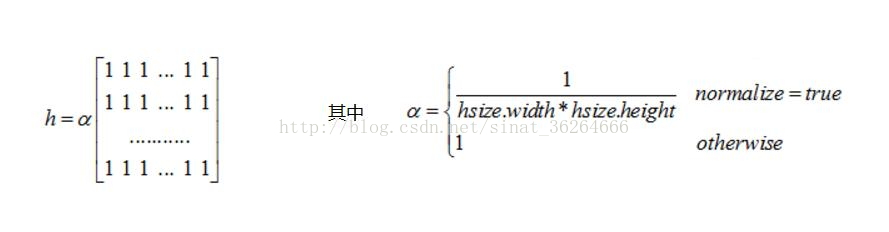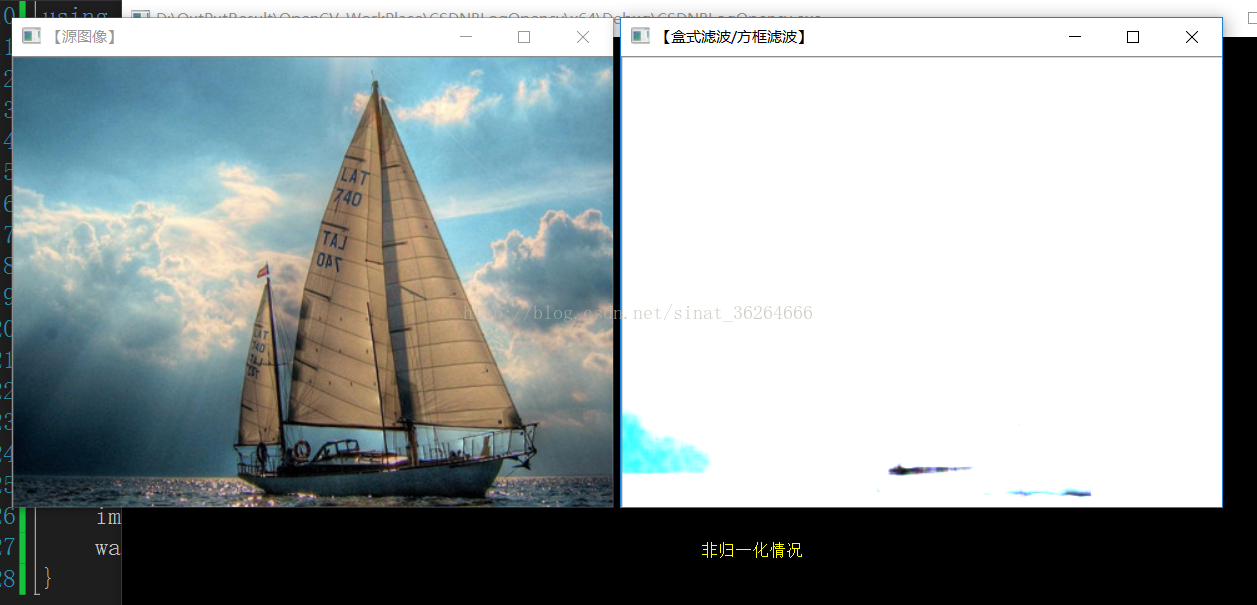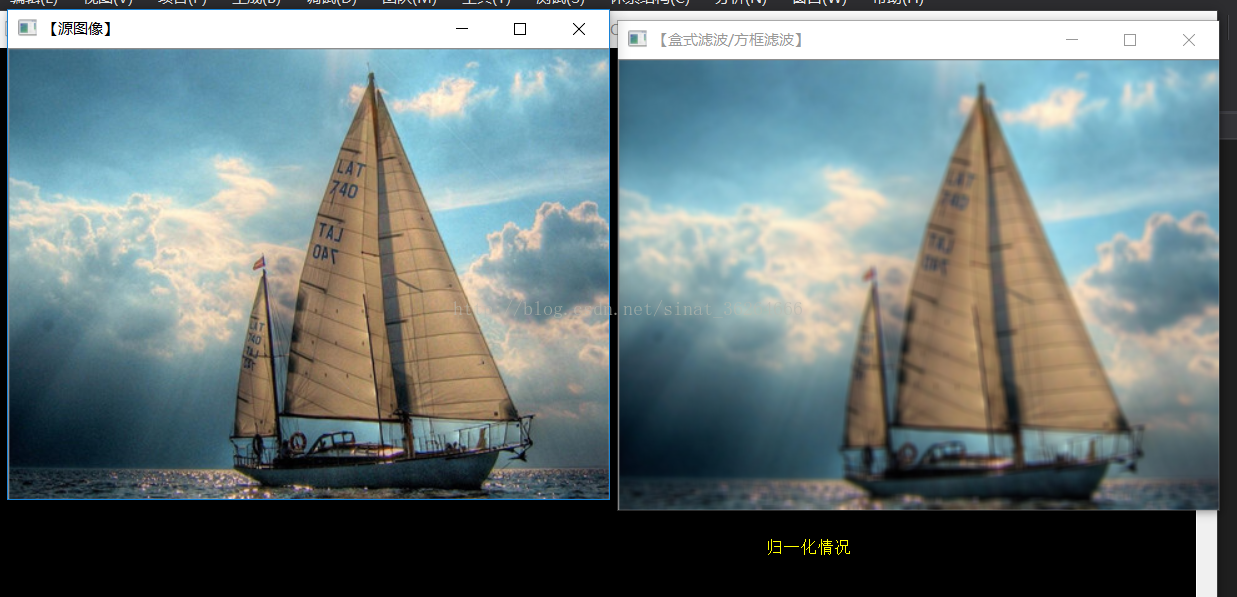可以发现，非归一化的时候，得到图像就是一片白色，对源图像毁坏太大，根本无法使用。

2、均值滤波

blur()函数——均值滤波

OpenCV将均值滤波封装在blur()函数中，作用是输入一副图像对其进行均值滤波。感兴趣的同学可以看看其源代码。下面来看下blur()函数的定义：

void blur( InputArray src, OutputArray dst, Size ksize, Point anchor=Point(-1,-1), int borderType=BORDER_DEFAULT );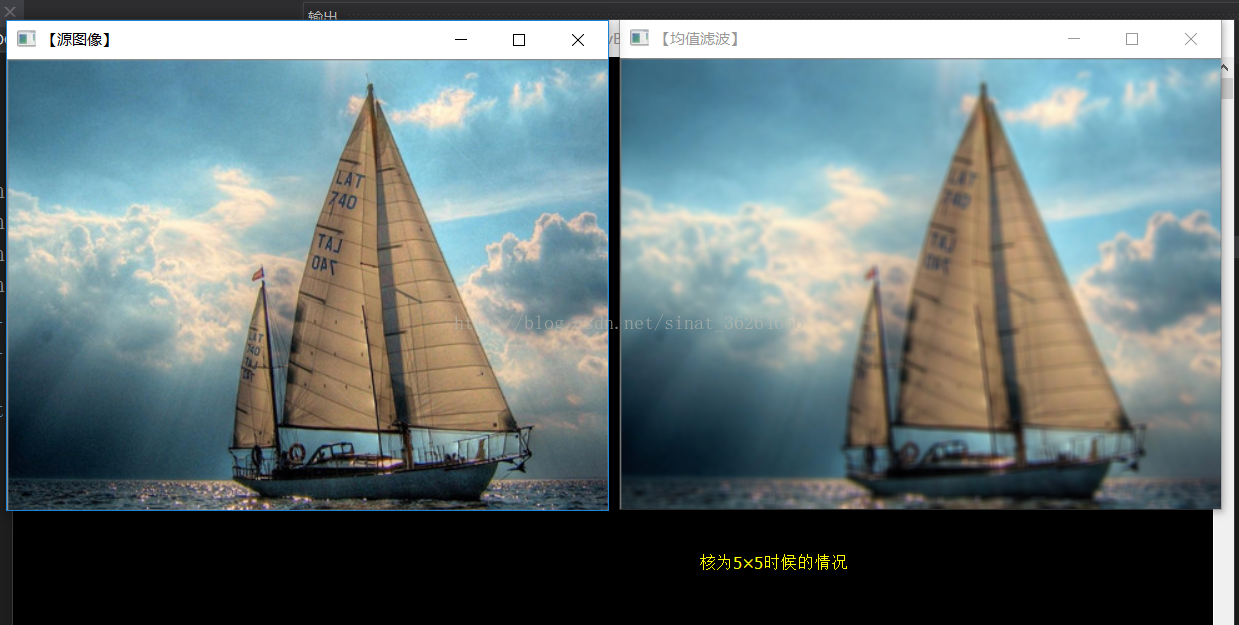blur()函数中的参数3，若核越大，则处理后的图像越模糊。上一节讲的盒式滤波（方框滤波），若将boxFilter()函数中的参数6，设为true，则表现的就是均值滤波，即归一化后的方框滤波。

3、高斯滤波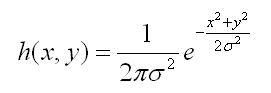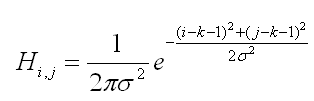“离散化”：上面已经提到了离散的高斯卷积核，实际上就是将高斯函数进行离散化，方便程序计算高斯模板；

“窗口”：这个窗口比喻得很形象，实际上我的理解就是M*N维的高斯模板；

“滑窗”：有了这个高斯模板，接下来是结合我们待处理的灰度数据，处理一个接着一个的像素点。例如说，处理完（1,1）这个点之后，接下来要处理（1,2）这个数据点，那么模板相当于右移了一个像素点，那么我们可以把这个过程形象地看作是滑窗。在碰上待处理图像的边缘时，往往处理不了，此时要进行特殊处理，比如说在检测到边缘时，复制原来的灰度数据，即不处理；

“卷积”：在高数里有过这个概念，然而在这个地方，卷积远远没有书上的那么复杂，实际上就是待处理图像的各像素灰度数据与模板对应元素的数值的加权和的运算过程，就称之为卷积。

GaussianBlur()函数——高斯滤波

OpenCV将高斯滤波封装在GaussianBlur()函数中，作用是输入一副图像对其进行高斯滤波。下面来看下GaussianBlur()函数的定义：

void GaussianBlur(InputArray src,OutputArray dst, Size ksize, double sigmaX, double sigmaY = 0, int borderType = BORDER_DEFAULT);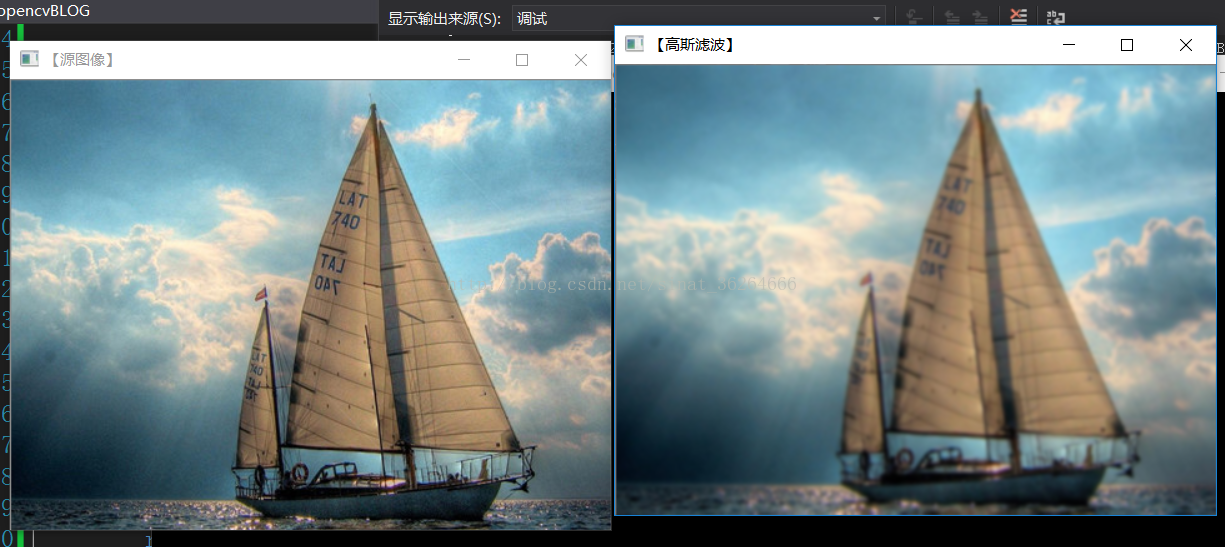4、中值滤波

medianBlur()函数——中值滤波
OpenCV将均值滤波封装在medianBlur()函数中，作用是输入一副图像对其进行中值滤波。下面来看下medianBlur()函数的定义：

void medianBlur( InputArray src, OutputArray dst, int ksize );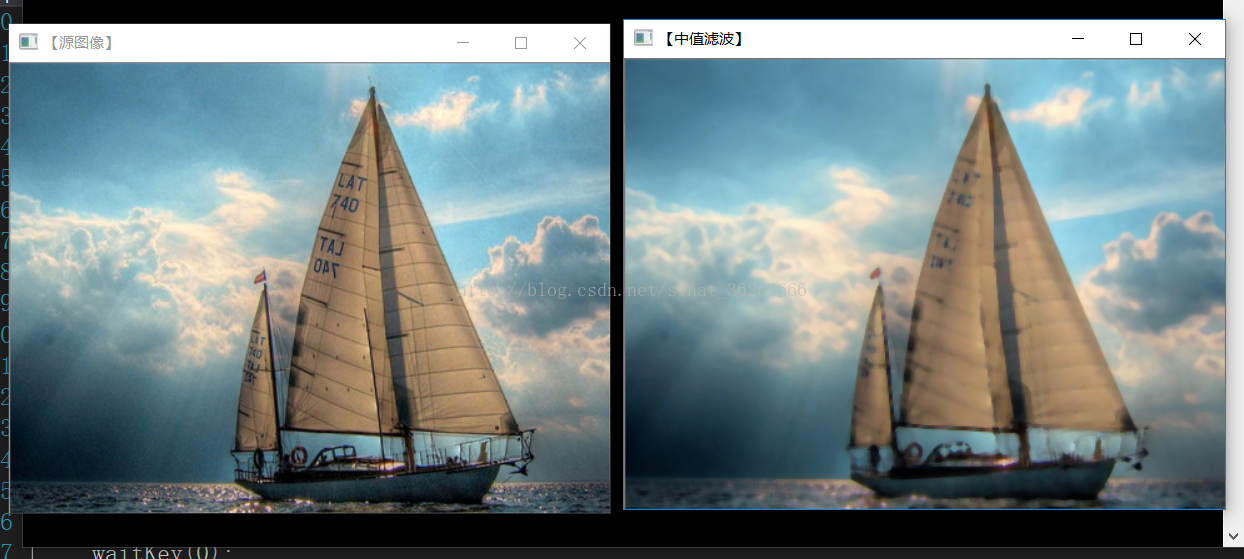可以发现，中值滤波可以很好保留物体的边缘信息。

5、双边滤波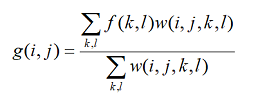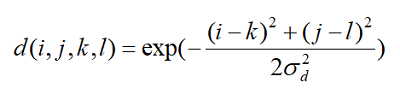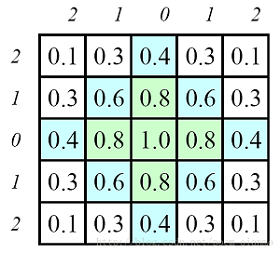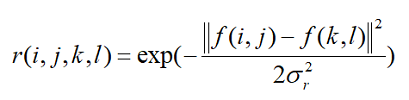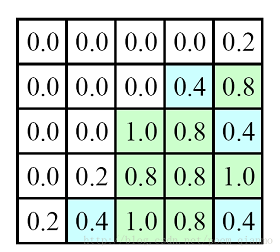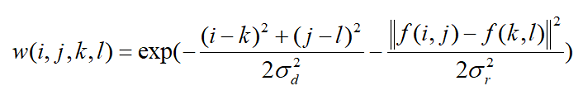bilateralFilter()——双边滤波

OpenCV将双边滤波封装在bilateralFilter()函数中，作用是输入一副图像对其进行双边滤波。下面来看下bilateralFilter()函数的定义：

void bilateralFilter( InputArray src, OutputArray dst, int d, double sigmaColor, double sigmaSpace, int borderType=BORDER_DEFAULT );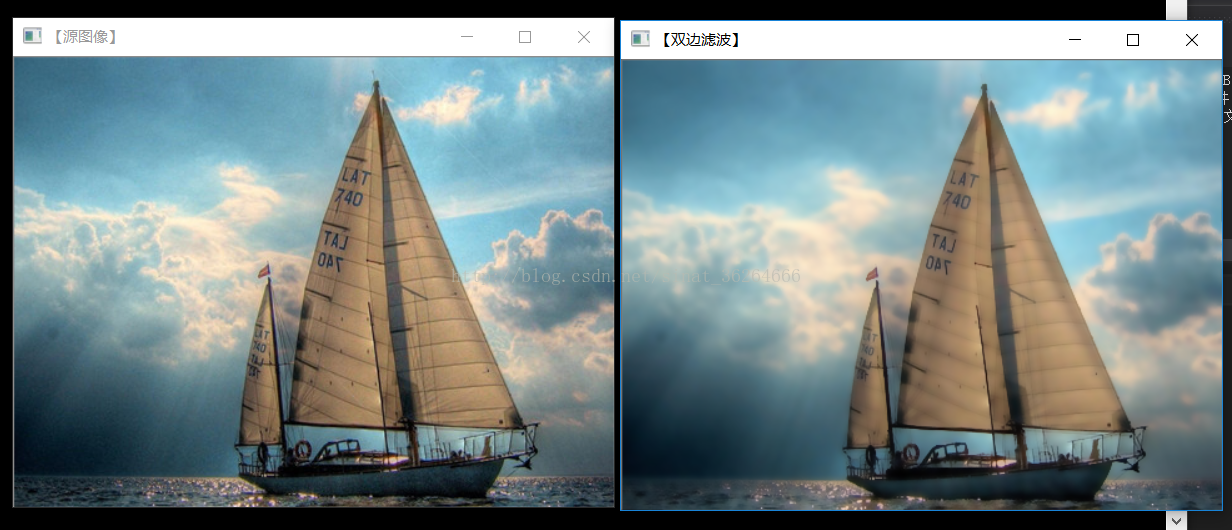可以发现双边滤波对图像物体的边缘信息保留的很完整。

2019-12-13 10:10:58 qq_17518433 阅读数 58
• ###### matlab信号处理和滤波去噪

739 人正在学习 去看看 刘昱显

1）滤波去噪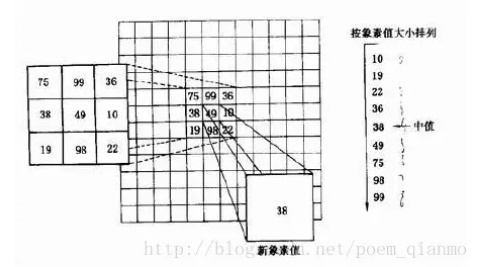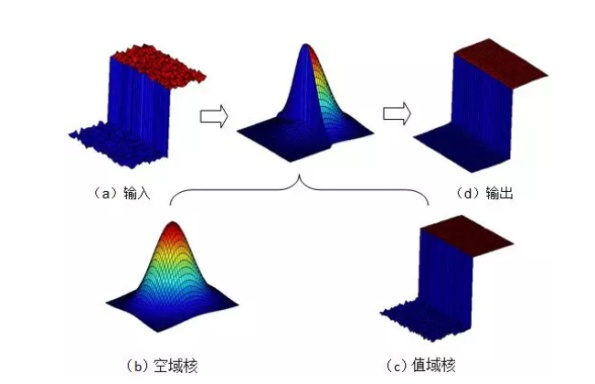2）连通域检测

0.首先设定speckleRange和speckleWindowSize，speckleWindowSize是指设置检测出的连通域中像素点个数，也就是连通域的大小。speckleRange是指设置判断两个点是否属于同一个连通域的阈值条件。

1.判断当前像素点四邻域的邻域点与当前像素点的差值diff，如果diff<speckleRange，则表示该邻域点与当前像素点是一个连通域，设置一个标记。然后再以该邻域点为中心判断其四邻域点，步骤同上。直至某一像素点四邻域的点均不满足条件，则停止。

2.步骤1完成后，判断被标记的像素点个数count，如果像素点个数count<=speckleWindowSize，则说明该连通域是一个小团块(blob)，则将当前像素点值设置为newValue(表示错误的视差值，newValue一般设置为负数或者0值)。否则，表示该连通域是个大团块，不做处理。同时建立标记值与是否为小团块的关系表rtype[label]，rtype[label]为0，表示label值对应的像素点属于小团块，为1则不属于小团块。

3.处理下一个像素点时，先判断其是否已经被标记：如果已经被标记，则根据关系表rtype[label]判断是否为小团块（blob），如果是，则直接将该像素值设置为newValue；如果不是，则不做处理。继续处理下一个像素。如果没有被标记，则按照步骤1处理。

4.所有像素点处理后，满足条件的区域会被设置为newValue值。

typedef cv::Point_<short> Point2s;
void myFilterSpeckles(cv::Mat &img, int newVal, int maxSpeckleSize, int maxDiff)
{
int width = img.cols;
int height = img.rows;
int imgSize = width * height;
int *pLabelBuf = (int*)malloc(sizeof(int)*imgSize);//标记值buffer
Point2s *pPointBuf = (Point2s*)malloc(sizeof(short)*imgSize);//点坐标buffer
uchar *pTypeBuf = (uchar*)malloc(sizeof(uchar)*imgSize);//blob判断标记buffer
//初始化Labelbuffer
int currentLabel = 0;
memset(pLabelBuf, 0, sizeof(int)*imgSize);

for (int i = 0; i < height; i++)
{
float *pData = img.ptr<float>(i);
int *pLabel = pLabelBuf + width * i;
for (int j = 0; j < width; j++)
{
if ((pData[j] - newVal) > 1e-1)
{
if (pLabel[j])
{
if (pTypeBuf[pLabel[j]])
{
pData[j] = newVal;
}
}
else
{
Point2s *pWave = pPointBuf;
Point2s curPoint(j, i);
currentLabel++;
int count = 0;
pLabel[j] = currentLabel;
while (pWave >= pPointBuf)
{
count++;
float *pCurPos = &img.at<float>(curPoint.y, curPoint.x);
float curValue = *pCurPos;
int *pCurLabel = pLabelBuf + width * curPoint.y + curPoint.x;
//bot
if (curPoint.y < height - 1 && !pCurLabel[+width] && pCurPos[+width] != newVal && abs(curValue - pCurPos[+width]) <= maxDiff)
{
pCurLabel[+width] = currentLabel;
*pWave++ = Point2s(curPoint.x, curPoint.y + 1);
}
//top
if (curPoint.y > 0 && !pCurLabel[-width] && pCurPos[-width] != newVal && abs(curValue - pCurPos[-width]) <= maxDiff)
{
pCurLabel[-width] = currentLabel;
*pWave++ = Point2s(curPoint.x, curPoint.y - 1);
}
//right
if (curPoint.x < width - 1 && !pCurLabel[+1] && pCurPos[+1] != newVal && abs(curValue - pCurPos[+1]) <= maxDiff)
{
pCurLabel[+1] = currentLabel;
*pWave++ = Point2s(curPoint.x + 1, curPoint.y);
}
//left
if (curPoint.x > 0 && !pCurLabel[-1] && pCurPos[-1] != newVal && abs(curValue - pCurPos[-1]) <= maxDiff)
{
pCurLabel[-1] = currentLabel;
*pWave++ = Point2s(curPoint.x - 1, curPoint.y);
}

--pWave;
curPoint = *pWave;
}

if (count <= maxSpeckleSize)
{
pTypeBuf[pLabel[j]] = 1;
pData[j] = (float)newVal;
}
else
{
pTypeBuf[pLabel[j]] = 0;
}
}
}
}
}

free(pLabelBuf);
free(pPointBuf);
free(pTypeBuf);
}


3)左右一致性检测

2019-10-26 17:34:40 wujuxKkoolerter 阅读数 147
• ###### matlab信号处理和滤波去噪

739 人正在学习 去看看 刘昱显

# 梯形高通滤波

$$H(u,v) = 1 - \begin{cases} 1 & D(u,v) \lt D_0 \\ \frac{D(u,v) - D_1}{D_0-D_1} & D_0 \lt D(u,v) \leq D_1 \\ 0 &D(u,v) > D_1 \end{cases} \tag{18-1} $$

Python语言实现的代码如下：

def trapezoidal_low_pass_kernel(img,D0=5,D1=10):
assert img.ndim == 2
r,c = img.shape,img.shape
u = np.arange(r)
v = np.arange(c)
u, v = np.meshgrid(u, v)
low_pass = np.sqrt( (u-r/2)**2 + (v-c/2)**2 )

idx = low_pass < D0
idx2 = (low_pass >= D0) & (low_pass <= D1)
idx3 = low_pass > D1

low_pass[idx] = 1
low_pass[idx2] = (low_pass[idx2] - D1) / (D1 - D0)
low_pass[idx3] = 0

return low_pass

def trapezoidal_high_pass_filter(img,D0=5,D1=15):
assert img.ndim == 2
gray = np.float64(img)
kernel = 1 - trapezoidal_low_pass_kernel(img,D0,D1)
gray_fft = np.fft.fft2(gray)
gray_fftshift = np.fft.fftshift(gray_fft)
dst = np.zeros_like(gray_fftshift)
dst_filtered = kernel * gray_fftshift
dst_ifftshift = np.fft.ifftshift(dst_filtered)
dst_ifft = np.fft.ifft2(dst_ifftshift)
dst = np.abs(np.real(dst_ifft))
dst = np.clip(dst,0,255)
return np.uint8(dst)